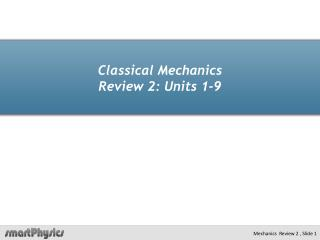DownloadDownload PresentationClassical Mechanics Review 2: Units 1-9

# Classical Mechanics Review 2: Units 1-9

Download Presentation## Classical Mechanics Review 2: Units 1-9

- - - - - - - - - - - - - - - - - - - - - - - - - - - E N D - - - - - - - - - - - - - - - - - - - - - - - - - - -
##### Presentation Transcript

1. Classical MechanicsReview 2: Units 1-9

2. Example: Work and Inclined planes • The block shown has a mass of 1.58 kg. The coefficient of friction is µk = 0.550 and θ = 20.0o. If the block is moved 2.25 mup the incline, calculate (including signs): • (a) the work done by gravity on the block; • (b) the work done on the block by the normal force; • (c) the work done by friction on the block. M q

3. Example: Block and spring 2.5 kg k=250 N/m h=1.5 m mk = 0.4 d = 0.50 m • A 2.5 kg box is released from rest 1.5 m above the ground and slides down a frictionless ramp. It slides across a floor that is frictionless, except for a small section 0.5 mwide that has a coefficient of kinetic friction of 0.2. At the left end, is a spring with spring constant 250 N/m. The box compresses the spring, and is accelerated back to the right. • What is the speed of the box at the bottom of the ramp? • What is the maximum distance the spring is compressed by the box?

4. Example: Block slides down the hill • A block of mass m starts from rest at the top of the frictionless, hemispherical hill of radius R. • (a) Find an expression for the block's speed when it is at an angle ϕ. • (b) Find the normal force N at that angle. • (c) At what angle does the block "fly off" the hill? • (d) With what speed will the block hit the ground?

5. Example: Pulley and Two Masses H = 2 m • H m2 = 2 kg • A block of mass m1 = 1 kgsits atop an inclined plane of angle θ = 20owith coefficient of kinetic friction 0.2 and is connected to mass m2 = 3 kg through a string that goes over a massless frictionless pulley. The system starts at rest and mass m2 falls through a height H = 2 m. • Use energy methods to find the velocity of mass m2 just before it hits the ground? What is the acceleration of the blocks? • m1 θ • m2

6. Example: Collision with a vertical spring • A vertical spring with k = 490 N/m is standing on the ground. A 1.0 kg block is placed at h =20 cm directly above the spring and dropped with an initial speed vi =5.0 m/s. • (a) What is the maximum compression of the spring? • (b) What is the position of the equilibrium of the block-spring system? 1kg vi k

7. Example: Pendulum L v Conserve Energy from initial to final position

8. Example: Pendulum T mg L

9. Example: Pendulum h h Conserve Energy from initial to final position.

10. Example: Pendulum r T mg h

11. Example: Blocks, Pulley and Spring • Two blocks m1 = 4 kg and m2 = 6 kg are connected by a string that passes over a pulley. m1 lies on an inclined surface of 20o and is connected to a spring of spring constant k = 120 N/m. The system is released from rest when the spring is unstretched. The coefficient of kinetic friction of the inclined surface and is 0.12. • Find the work of friction when m2 has fallen a distance D = 0.2 m. • Find the speed of the blocks at that time. • At what distance d does the acceleration of the blocks becomes zero? k m1 20o • 20o m2

12. Example: Popgun (Spring and Gravity) • The launching mechanism of a popgun consists of a spring. when the spring is compressed 0.120 m, the gun when fired vertically is able to launch a 35.0 g projectile to a maximum height of 20.0 m above its position as it leaves the spring. • (a) Determine the spring constant • (b) Find the speed of the projectile as it • moves through the equilibrium position • of the spring.

13. Example: Spring and Mass • A spring is hung vertically and an object of mass m is attached to its lower end. Under the action of the load mg the spring stretches a distance d from its equilibrium position. • 1. If a spring is stretched 2.0 cm by a suspended object of mass 0.55 kg what is the spring constant k? •   2. How much work is done by the spring on the object as it stretches through this distance?